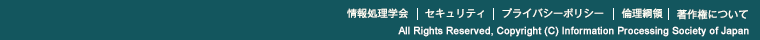5A-01
The LR-dispersion problem
○赤木俊裕（群馬大），荒木徹也（NII），中野眞一（群馬大）
In this paper we design two algorithms for some variants of the dispersion problem.
Given a set P of points on the horizontal line and an integer k we wish to find a subset S of P such that |S| = k and maximizing the cost min{cost(s)}, where cost(s) is the sum of distances to its left neighbour and right neighbour in S. (For the leftmost point in S its cost is the distance to its right neighbour. Similar for the rightmost points.) The problem is called the LR- dispersion problem. In this paper we give O(n log n) greedy algorithms for the LR-dispersion problem and one more variant of the dispersion problem.